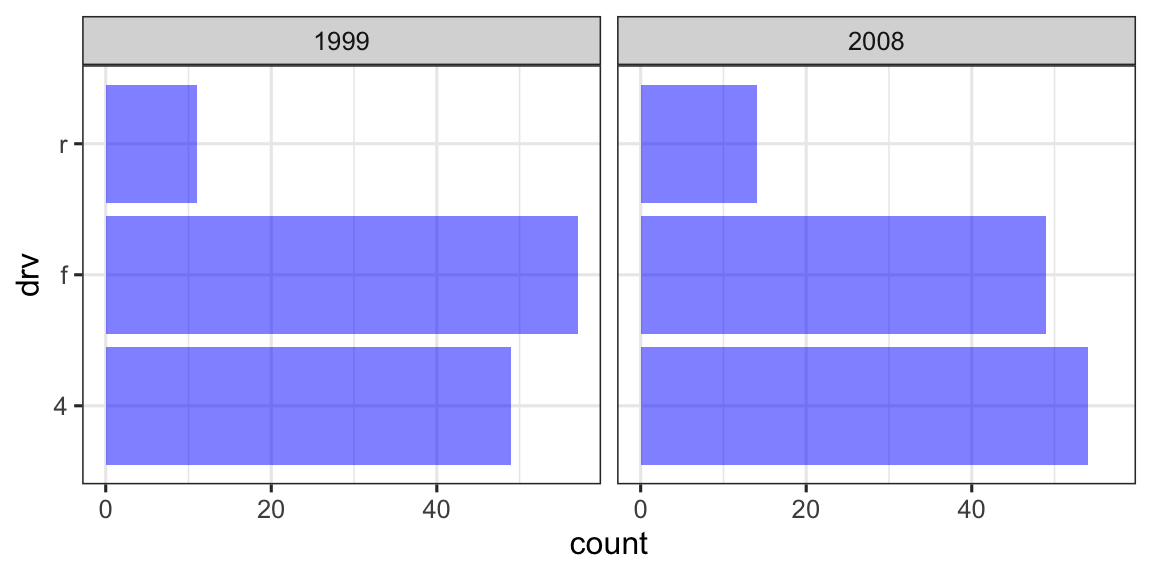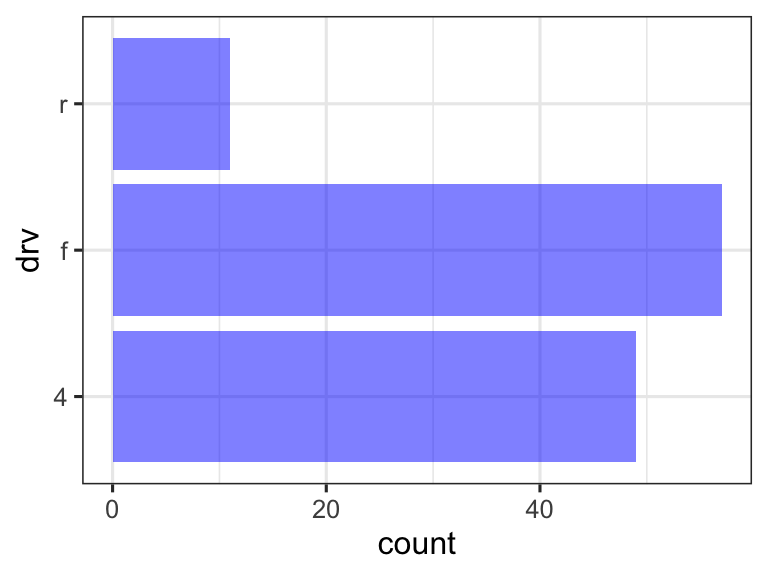## In-class worksheet 30

May 9, 2019

In this worksheet, we will use the libraries tidyverse, sf, and gganimate:

``````library(tidyverse)
theme_set(theme_bw(base_size=12)) # set default ggplot2 theme
library(sf)
library(gganimate)``````

## 1. A grammar of animation

The gganimate package provides a rich grammar of animation. The key idea is that animations are similar to faceting, only that we facet in time rather than in space. For example, consider this plot, which shows the distribution of drive trains in cars from 1999 and 2008.

``````ggplot(mpg, aes(drv)) +
geom_bar(fill = "blue", alpha = 0.5) +
coord_flip() +
facet_wrap(~year)``````We can animate this plot by replacing `facet_wrap()` with `transition_states()`. Everything else remains the same.

``````ggplot(mpg, aes(drv)) +
geom_bar(fill = "blue", alpha = 0.5) +
coord_flip() +
transition_states(year) # we don't need a ~ here before year``````However, what is missing from this animation now is that we don’t know which frames belong to which year. We can resolve this issue by adding a plot title. The string `{closest_state}` inside the title will be replaced by the value of the variable on which we are transitioning.

``````ggplot(mpg, aes(drv)) +
geom_bar(fill = "blue", alpha = 0.5) +
coord_flip() +
labs(title = "Year: {closest_state}") +
transition_states(year)``````